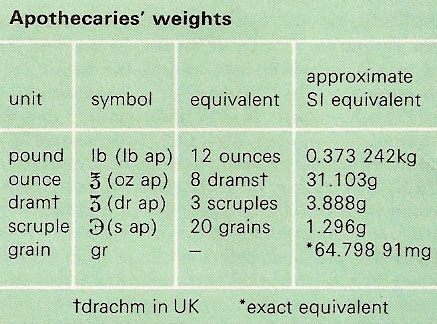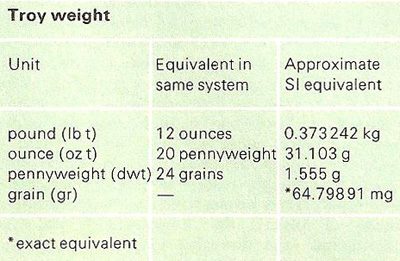A# units of massFigire 1. Apothecaries' weights.Figire 2. Troy weight.

## apothecaries' weights

Apothecaries' weights is a system of weights formerly used by pharmacists in preparing medicines (see Figure 1).

1 oz. ap = 1 oz. troy = 1.0971 oz. avoirdupois = 31.103 grams.

## avoirdupois

Avoirdupois is the system of weights customarily used in the US (and formerly in the UK) for most goods except gems and drugs for which are employed respectively troy weights and apothecaries weights. There are 7,000 grains or 16 ounces in the pound avoidupois. The term is a corruption of French, meaning "property of weight".)

## carat

A carat is a unit of mass used for weighing precious stones. Since 1913 the internationally accepted carat has been the metric carat (CM) of 200 milligrams. The purity of gold is also expressed in carats (usually spelled "karat" in the United States). Here one karat is a twenty-fourth part; thus, pure gold is 24-karat; "18-karat gold" contains 75% gold and 25% other noble metals, and so on.

## grain

A grain (gr) is the fundamental US (and formerly British) unit of mass, shared between the avoirdupois, troy, and apothecaries' systems. The Imperial grain is defined equal to 0.064 79891 grams exactly.

## gram

A gram (g) is the fundamental unit of mass in the CGS version of the metric system. It approximates to the mass of a cubic centimeter of water. 1,000 grams = 1 kilogram.

## hundredweight

A hundredweight (cwt) is the name of two units of mass. In the US the short hundredweight of 100 pounds is used. The Imperial (long) hundredweights is 112 pounds.

## kilogram

The kilogram (kg) is the base unit of mass in the SI system. 1 kg = 1,000 g = 2.20462 lb. The kilogram is the only SI unit that is defined in terms of an artifact instead of an experiment, this artifact being a cylindrical platinum-iridium slug kept by the International Bureau of Weights and Measures at Sèvres, near Paris. It is copied by all the bureaus of standards and laboratories in the world which adhere to the SI. For example, a replica of the metal cylinder at Sèvres is kept at the National Institute of Standards and Technology in Gaithersburg, Maryland, and serves as the SI standard of mass for the United States.

The kilogram used to be defined as the mass of 1 liter of water. This mass served as the archetype for the artifact at Sèvres.

## ounce

The ounce (oz) is a unit of mass in the apothecaries', avoirdupois, and troy systems. 1oz ap = 1oz troy = 1.0971oz avoirdupois = 31.103 grams.

## pound

The pound (lb) is the name of various units of mass. The pound avoirdupois is defined as being exactly 0.45359237 kilogram. The "metric pound" commonly used in continental Europe is 500 grams. A stone is a UK unit of weight equal to 14 pounds.

## ton

A ton is any of various actual or nominal units of mass. The short ton commonly used in the US is 2,000 pounds, the long ton is 2,240 pounds. The metric ton or tonne (t) is 1,000 kilograms (2,204.62 pounds). The ton used for measuring ships' cargoes is 40 cubic feet in the US, 42 cubic feet in the UK. The register ton used for describing the capacity of merchant ships is 100 cubic feet; displacement ton used for describing warships (equivalent to 35 cubic feet of sea water) refers to the weight of water displaced by the ship – and hence to the actual weight of the ship.

## troy weight

Troy weight is a system of weights used for precious metals and stones, named for the French town of Troyes, famed for its medieval trade fairs (see Figure 2). Today the price of gold is still quoted in dollars per ounce. The troy ounce, equivalent to 1.0971 ounces avoirdupois, is equal to the apothecaries' ounce.